## Doing Excel Things in R #4

We are going to examine some data from a petroleum refinery in R using the petrol data file from the MASS package. This is basically an exercise in descriptive statistics.

Here’s the code!

library(MASS)

It helps to have a visual of the structure and headings of the data.

str(petrol)
## 'data.frame':    32 obs. of  6 variables:
##  $No : Factor w/ 10 levels "A","B","C","D",..: 1 1 1 1 2 2 2 3 3 3 ... ##$ SG : num  50.8 50.8 50.8 50.8 40.8 40.8 40.8 40 40 40 ...
##  $VP : num 8.6 8.6 8.6 8.6 3.5 3.5 3.5 6.1 6.1 6.1 ... ##$ V10: int  190 190 190 190 210 210 210 217 217 217 ...
##  $EP : int 205 275 345 407 218 273 347 212 272 340 ... ##$ Y  : num  12.2 22.3 34.7 45.7 8 13.1 26.6 7.4 18.2 30.4 ...
head(petrol)
##   No   SG  VP V10  EP    Y
## 1  A 50.8 8.6 190 205 12.2
## 2  A 50.8 8.6 190 275 22.3
## 3  A 50.8 8.6 190 345 34.7
## 4  A 50.8 8.6 190 407 45.7
## 5  B 40.8 3.5 210 218  8.0
## 6  B 40.8 3.5 210 273 13.1

For purposes of understanding what we are doing with the data, the different columns refer to:

1. SG = specific gravity in degrees API
2. VP = vapor pressure (psi)
3. V10 = volatility of crude oil, ASTM 10% point
4. EP = desired volatility of gasoline
5. Y = yield as percentage of crude

We can find the mean of each column one at a time:

mean(petrol$SG) ##  39.25 mean(petrol$VP)
##  4.18125
mean(petrol$V10) ##  241.5 mean(petrol$EP)
##  332.0938
mean(petrol$Y) ##  19.65938 Can we find all column means more simply? Yes. But we can’t use the colMeans command colMeans(petrol, na.rm = FALSE, dims = 1) because our petrol data have a non-numeric column, No (see the head command above). So we have to remove the non-numeric column. When we do, the colMeans command works just fine. petrol$No <- NULL
colMeans(petrol, na.rm = FALSE, dims = 1)
##        SG        VP       V10        EP         Y
##  39.25000   4.18125 241.50000 332.09375  19.65938

Another way would be to use the apply command.

apply(petrol[, c('SG','VP', 'V10', 'EP', 'Y')], 2, mean)
##        SG        VP       V10        EP         Y
##  39.25000   4.18125 241.50000 332.09375  19.65938

Finding the mode is not as simple in R. In R, the mode command refers to the storage mode of the object.

mode(petrol$SG) ##  "numeric" Not what we want, so we have to back our way into it. First, create an object and then assign a table to it. modeSG <- table(petrol$SG)
modeSG
##
## 31.8 32.2 38.1 38.4   40 40.3 40.8 41.3 50.8
##    3    5    3    4    3    3    3    4    4

Looking at the output, we can see which value appears the most. But we can use R to find the maximum value in the table. That’s the mode we are looking for.

max(modeSG)
##  5

Here, we see the value 32.2 shows up five times in the SG column, so that is our mode.

We can do that again to find modes one by one.

modeVP <- table(petrol$VP) modeVP ## ## 0.2 1.2 1.8 2.4 3.5 4.8 5.2 6.1 8.6 ## 3 3 4 2 3 3 3 7 4 max(modeVP) ##  7 modeV10 <- table(petrol$V10)
modeV10
##
## 190 210 217 220 231 236 267 274 284 316
##   4   3   3   4   3   3   4   3   2   3
max(modeV10)
##  4
modeEP <- table(petrol$EP) modeEP ## ## 205 212 218 235 267 272 273 275 285 300 307 340 345 347 351 358 360 365 ## 1 1 1 2 1 1 1 2 1 1 1 1 1 1 1 1 1 3 ## 367 379 395 402 407 410 416 424 428 444 ## 1 1 1 1 1 1 1 1 1 1 max(modeEP) ##  3 modeY <- table(petrol$Y)
modeY
##
##  2.8    5  6.4  6.9  7.4    8  8.5   10 12.2 13.1   14 14.4 14.7 15.2 16.1
##    1    1    1    1    1    1    1    1    1    1    1    1    1    1    1
## 17.6   18 18.2 22.3 23.2 24.8   26 26.6 26.8 27.8 30.4 31.7 32.1 33.6 34.7
##    1    1    1    1    1    1    1    1    1    1    1    1    1    1    1
## 34.9 45.7
##    1    1
max(modeY)
##  1

You get the idea…

Likewise, we can find the median value for each column, one by one.

median(petrol$SG) ##  40 median(petrol$VP)
##  4.8
median(petrol$V10) ##  231 median(petrol$EP)
##  349
median(petrol$Y) ##  17.8 Or we can use the apply command to find them all at once. apply(petrol[, c('SG','VP', 'V10', 'EP', 'Y')], 2, median) ## SG VP V10 EP Y ## 40.0 4.8 231.0 349.0 17.8 It really is a matter of personal taste. My son says the way you code shows your “coding accent.” Every coder has a different accent. One way isn’t better than another, just different. Let’s find the range of our data. First, the one-at-a-time method. range(petrol$SG)
##  31.8 50.8

Or we can use the apply command.

apply(petrol[, c('SG','VP', 'V10', 'EP', 'Y')], 2, range)
##        SG  VP V10  EP    Y
## [1,] 31.8 0.2 190 205  2.8
## [2,] 50.8 8.6 316 444 45.7

We can find the standard deviations using the sd and apply commands.

sd(petrol$SG) ##  5.635429 apply(petrol[, c('SG','VP', 'V10', 'EP', 'Y')], 2, sd) ## SG VP V10 EP Y ## 5.635429 2.619830 37.541375 69.755961 10.722417 We can calculate the variance in different ways, as well. Square the sd command or just use the var command. sd(petrol$SG)^2
##  31.75806
var(petrol$SG) ##  31.75806 Enough data. Let’s try some graphs. We can draw boxplots for data summaries. This is pretty easy in R. Here is the basic version. Boxplots show the minimum, first quartile, median, third quartile, and maximum values, with any outliers. boxplot(petrol$EP)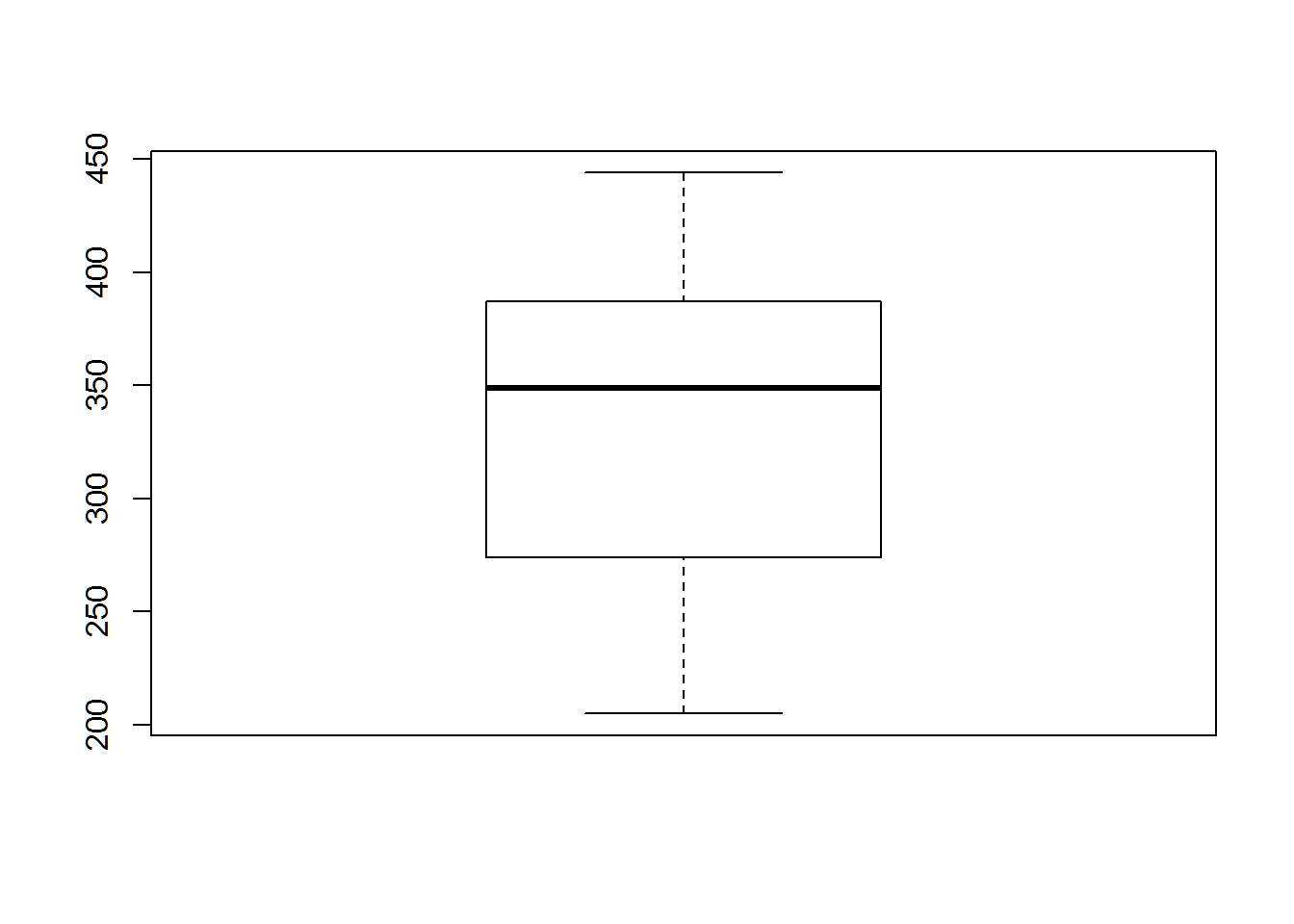It shows the median is 349, which we saw earlier, and the range from 205 to 444.

We can jazz the boxplot up a bit, adding color and labels.

boxplot(petrol$EP, ylab = 'EP', col = 'blue')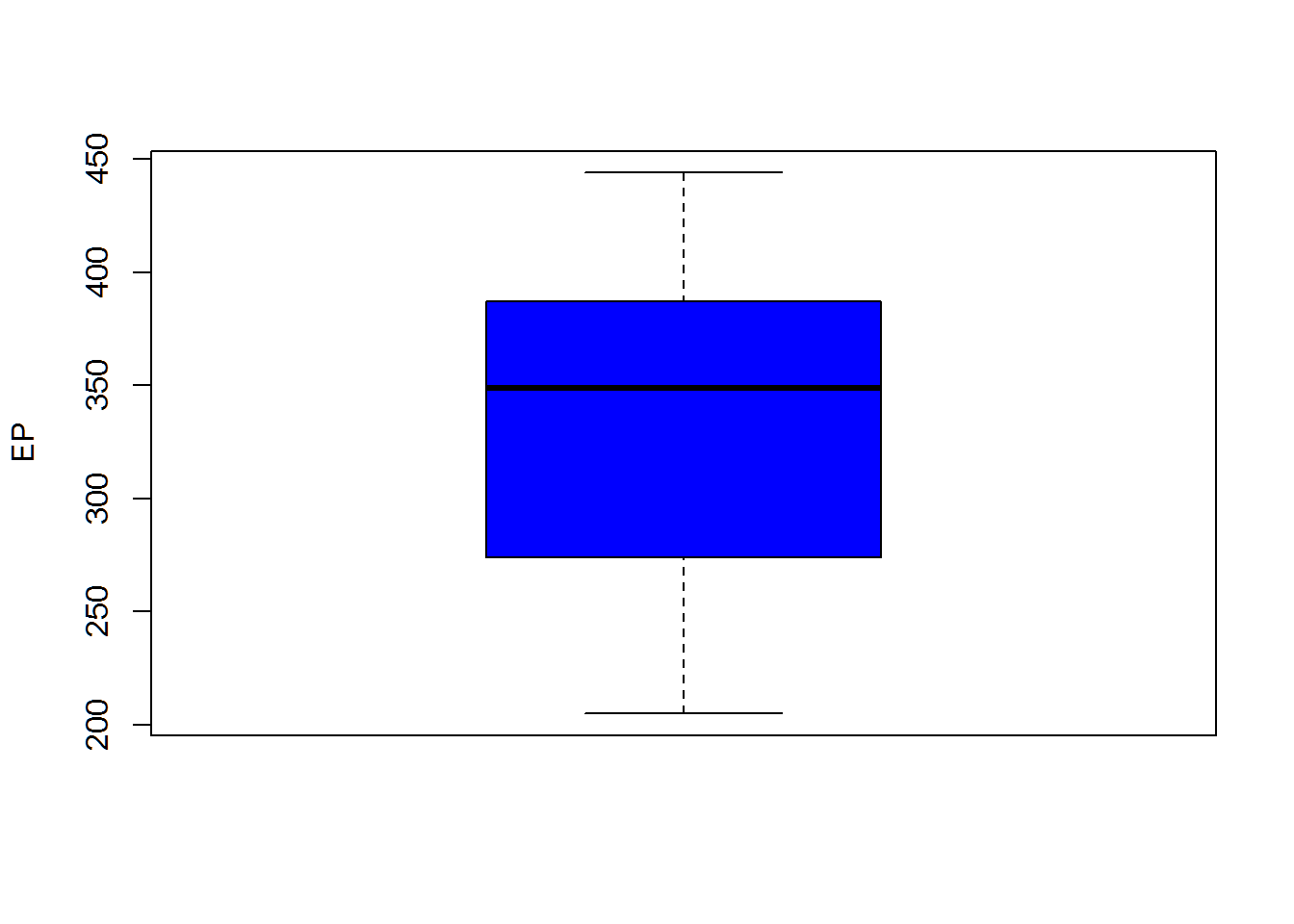Another useful graph for descriptive statistics is the histogram. First, we build a basic histogram of EP values. hist(petrol$EP)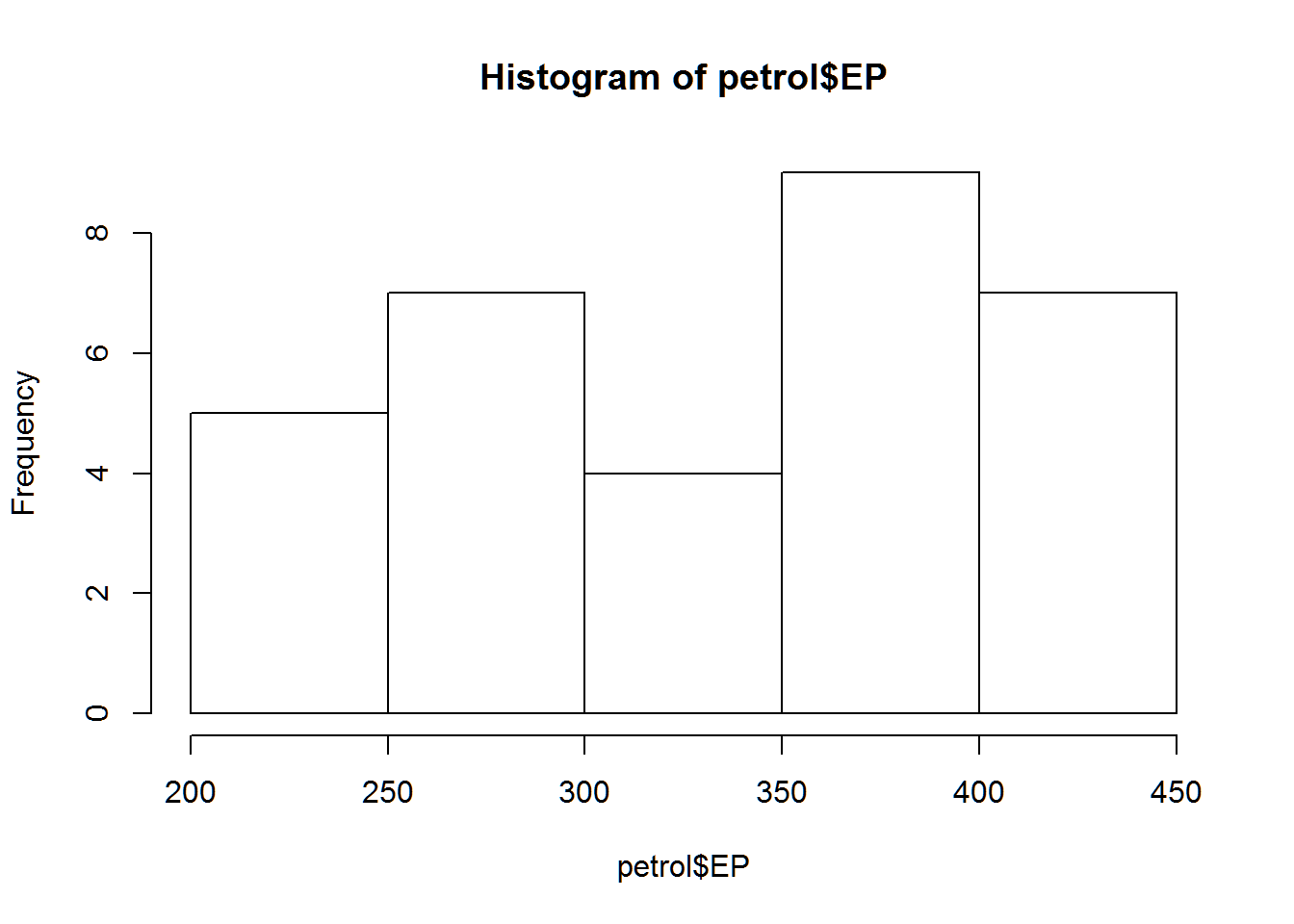It might be helpful to add labels and color. We can also define the number of bins and start the x-axis at the origin (zero) to get a better picture of the distribution of values. Try using different values for breaks. I used 10, 15, and 20 and settled on 15.

hist(petrol$EP, xlab = 'Desired Volatility of Gasoline', col = 'red', breaks = 15, main = 'Gas Volatility', xlim = c(0,500))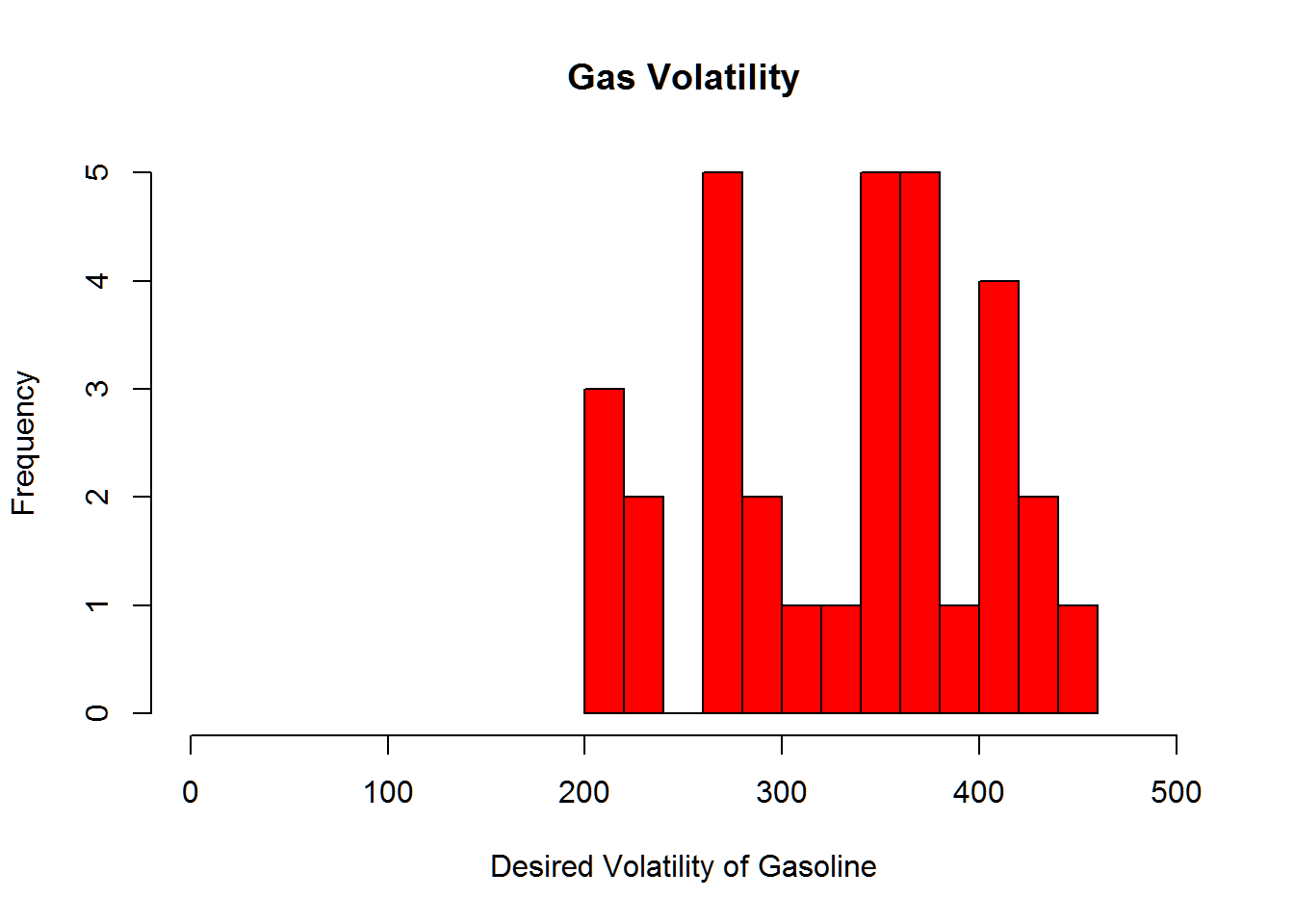So far, we have been interested in describing the statistics. But we use R (and Excel) to identify relationships between variables. Here is a neat graphic I haven’t seen in Excel. plot(petrol)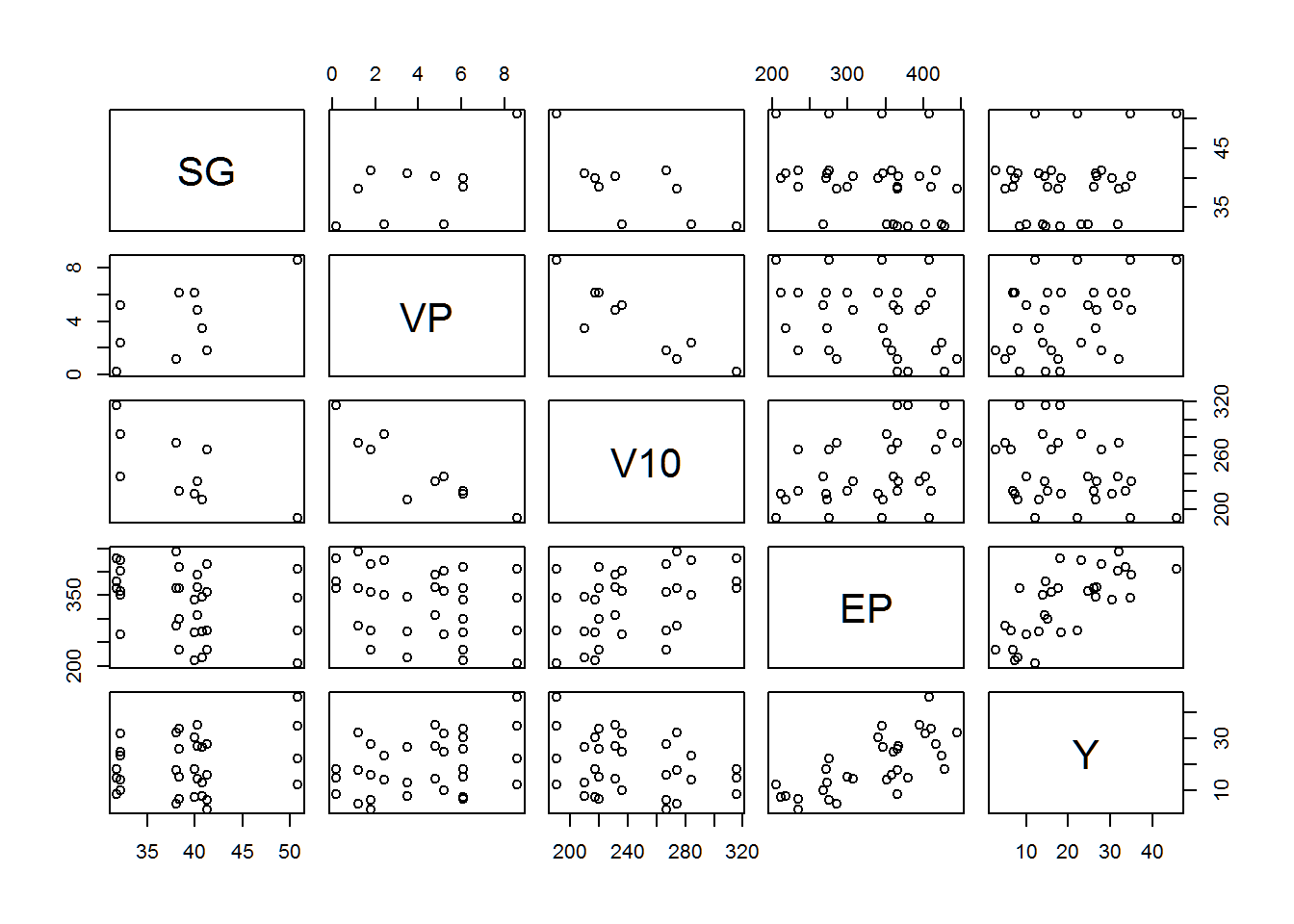What we have done here is plot each variable in the petrol file against every other variable. Remember, we removed the No column earlier from the file so it does not appear in these graphs. If the No column were still in the petrol data, we could have done the same thing with this command: plot(petrol[, 2:6]). Now we can begin to ask about relationships within the data. By examining the plots, it looks like there might be some kind of relationship between VP and V10. There might also be something going on between EP and Y. One way to test this quickly is to look for correlations. We all know “Correlation is not causation” but it can still be helpful in identifying possible relationships. Let’s look for the correlation between VP and V10. cor.test(petrol$VP, petrol$V10, method = 'pearson') ## ## Pearson's product-moment correlation ## ## data: petrol$VP and petrol$V10 ## t = -11.74, df = 30, p-value = 9.628e-13 ## alternative hypothesis: true correlation is not equal to 0 ## 95 percent confidence interval: ## -0.9535892 -0.8150977 ## sample estimates: ## cor ## -0.9062248 We can see the order of the variables doesn’t matter with correlations. That is, we don’t need to know which variable is dependent (the y-variable) and which one is independent (the x-variable) at this point. It will matter later, though, when we are trying to find a function for the variables. cor.test(petrol$V10, petrol$VP, method = 'pearson') ## ## Pearson's product-moment correlation ## ## data: petrol$V10 and petrol$VP ## t = -11.74, df = 30, p-value = 9.628e-13 ## alternative hypothesis: true correlation is not equal to 0 ## 95 percent confidence interval: ## -0.9535892 -0.8150977 ## sample estimates: ## cor ## -0.9062248 In both cases, the correlation is -0.906, which is pretty good. Now for the correlation between Y and EP. cor.test(petrol$EP, petrol$Y, method = 'pearson') ## ## Pearson's product-moment correlation ## ## data: petrol$EP and petrol$Y ## t = 5.5463, df = 30, p-value = 4.983e-06 ## alternative hypothesis: true correlation is not equal to 0 ## 95 percent confidence interval: ## 0.4825569 0.8494640 ## sample estimates: ## cor ## 0.7115262 The correlation here is 0.712. So our intuitive sense was proven correct. We could make life easier for ourselves if we were just to create a correlation table, which shows the correlations between all the variables. Remember, a variable correlated to itself has a correlation of 1. We need to load the corrplot package to create the table graphically, but we can do a basic table in R easily enough. Pay attention to how the table is structured. library(corrplot) M <- cor(petrol) M ## SG VP V10 EP Y ## SG 1.0000000 0.6205867 -0.7001539 -0.3216782 0.2463260 ## VP 0.6205867 1.0000000 -0.9062248 -0.2979843 0.3840706 ## V10 -0.7001539 -0.9062248 1.0000000 0.4122466 -0.3150243 ## EP -0.3216782 -0.2979843 0.4122466 1.0000000 0.7115262 ## Y 0.2463260 0.3840706 -0.3150243 0.7115262 1.0000000 The graphic representation is more interesting and resembles a heat map. corrplot(M, method = 'number')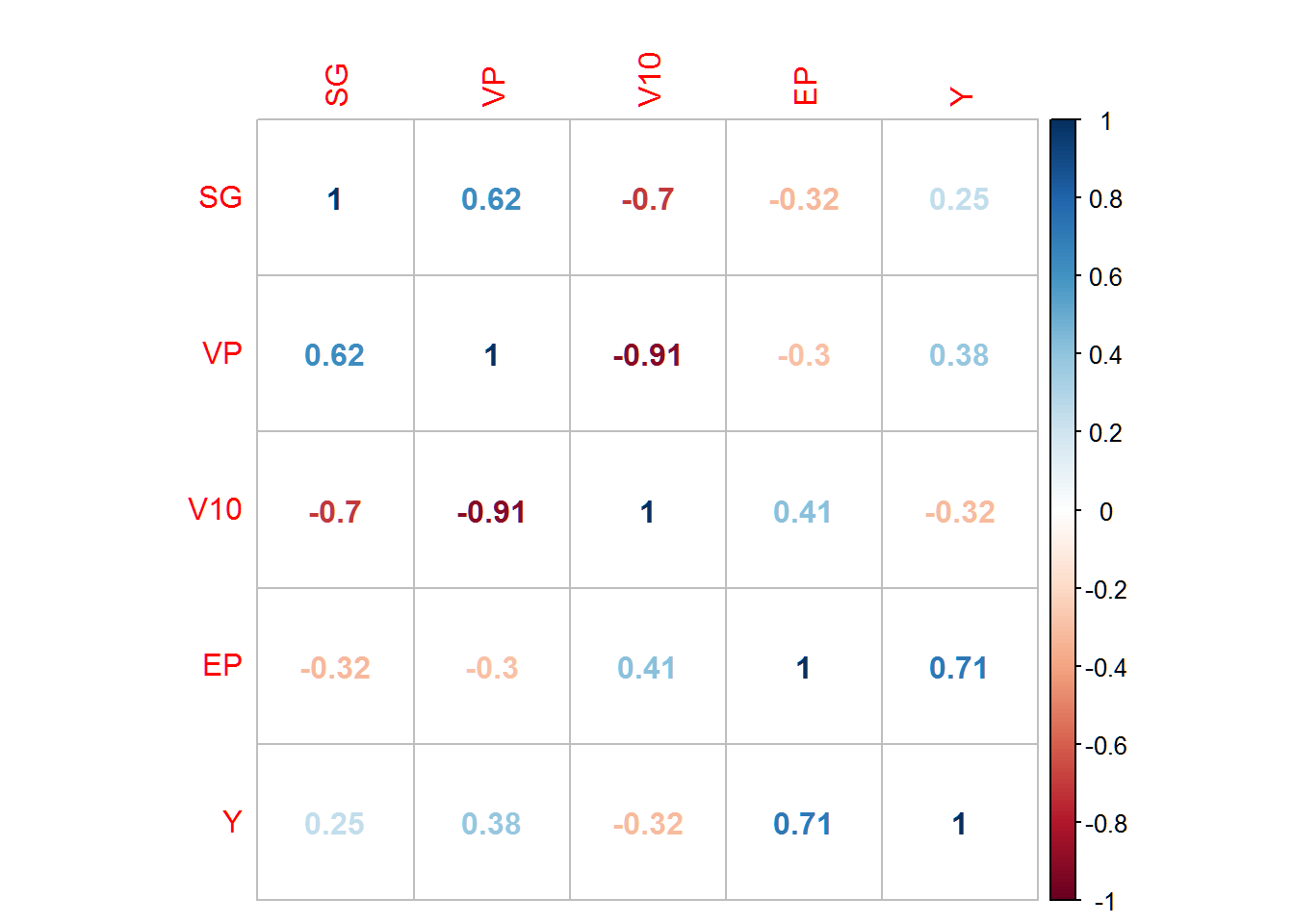The corrplot command contains numerous methods for representing correlation values, so explore them on your own to see which suits your needs best. If we use a rule that we are interested in correlation values between 0.60 and 1 or between -0.60 and -1 we can see there are relationships of interest between the following pairs: VP and SG; V10 and SG; V10 and VP; and Y and EP. Let’s make simple linear regression models between these variables to define functions that describe the relationship between the data. Now, we need to determine which variable in the pair is independent (x-variable) and which variable is dependent (y-variable). I am not a petroleum engineer, but I am guessing that pressure depends on volatility. If I am wrong about this, please let me know and I will fix this. But for illustrative purposes only, here is how to create linear models in R using the lm command. regV10.VP <- lm(VP ~ V10, data = petrol) regV10.VP ## ## Call: ## lm(formula = VP ~ V10, data = petrol) ## ## Coefficients: ## (Intercept) V10 ## 19.45396 -0.06324 So the equation for VP would be VP = -0.06324(V10) + 19.45396 where V10 is the x-variable and VP is the y-variable. We can create a plot of the data with our linear function included. First, we plot VP against V10. We have to define the length of the x- and y-axes to make this work. We add the regression line using the abline command. plot(petrol$V10, petrol$VP, xlim = c(0, 320), ylim = c(0, 20), xlab = 'V10-Crude Volatility', ylab = ' VP-Vapor Pressure', main = 'Vapor vs. Volatility') abline(regV10.VP)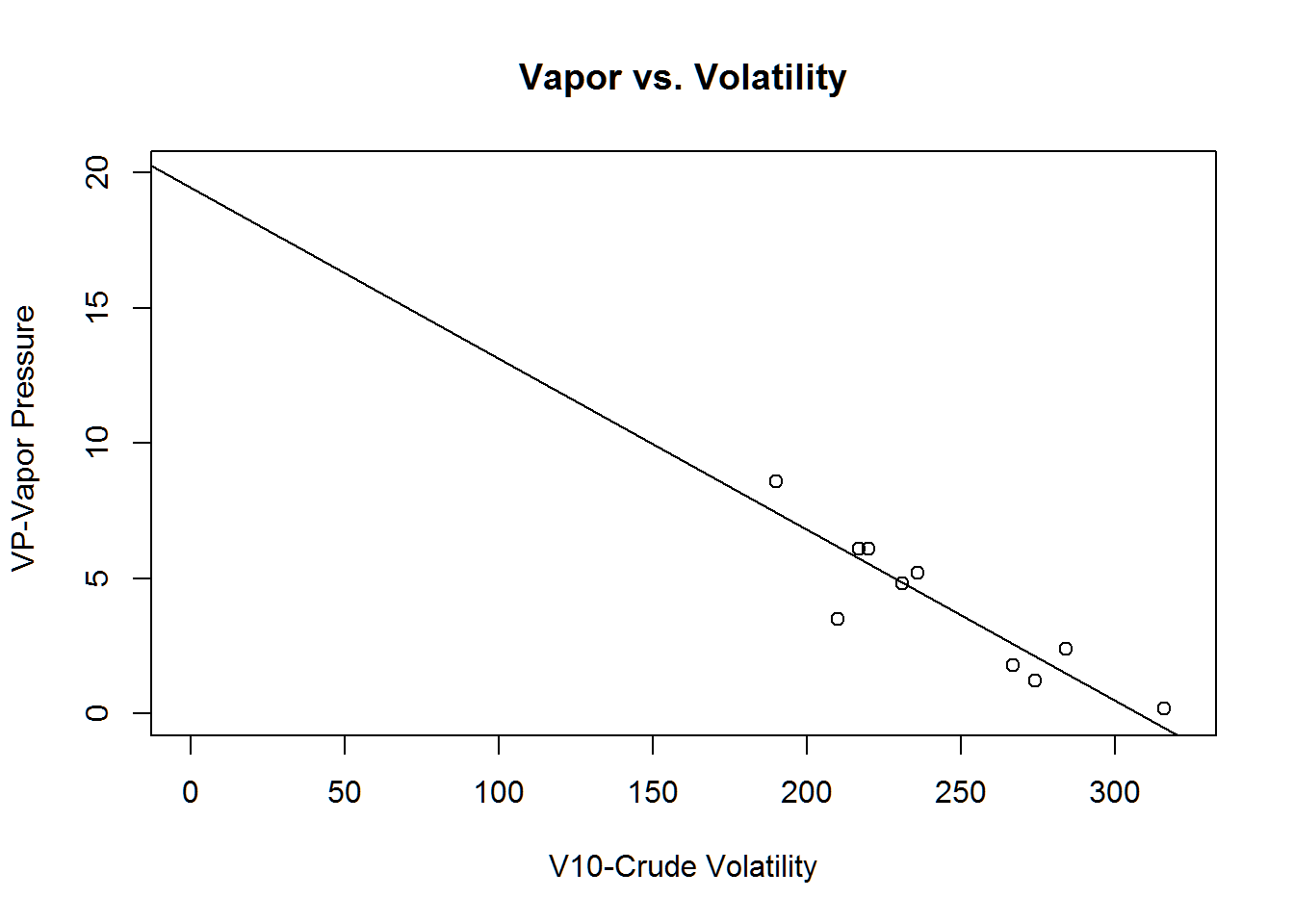We can do the same linear regression for each variable pair, matching them one-to-one. Remember, you can use the graphic from the plot(petrol) command above to determine the appropriate limits for the x- and y-axes. regSG.VP <- lm(VP ~ SG, data = petrol) regSG.VP ## ## Call: ## lm(formula = VP ~ SG, data = petrol) ## ## Coefficients: ## (Intercept) SG ## -7.1424 0.2885 plot(petrol$SG, petrol$VP, xlim = c(0, 60), ylim = c(-10, 10), xlab = 'SG-Specific Gravity', ylab = ' VP-Vapor Pressure', main = 'Pressure vs Gravity') abline(regSG.VP)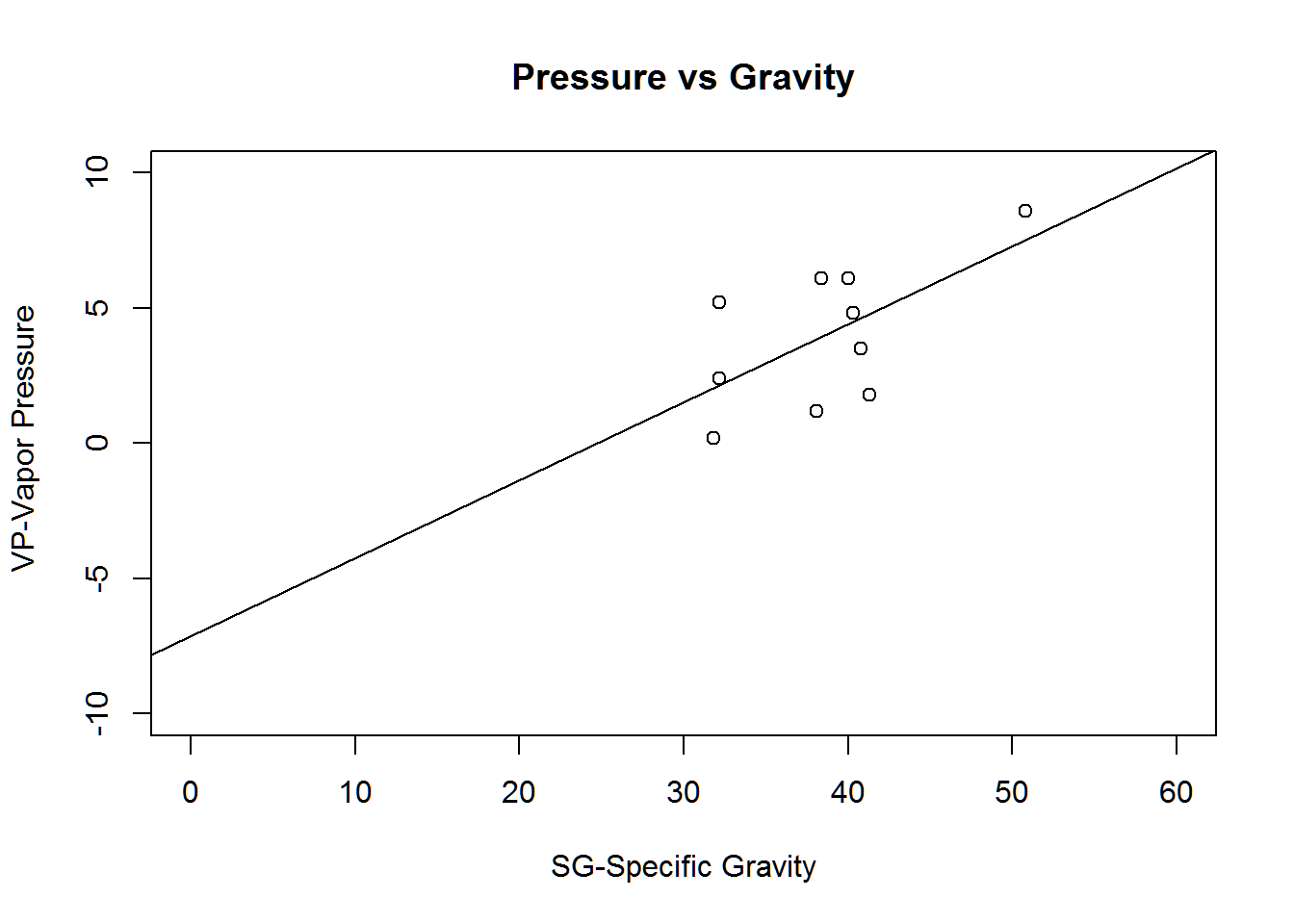regSG.V10 <- lm(V10 ~ SG, data = petrol) regSG.V10 ## ## Call: ## lm(formula = V10 ~ SG, data = petrol) ## ## Coefficients: ## (Intercept) SG ## 424.570 -4.664 plot(petrol$SG, petrol$V10, xlim = c(0, 60), ylim = c(0, 430), xlab = 'SG-Specific Gravity', ylab = 'V10-Crude Volatility', main = 'Volatility vs Gravity') abline(regSG.V10)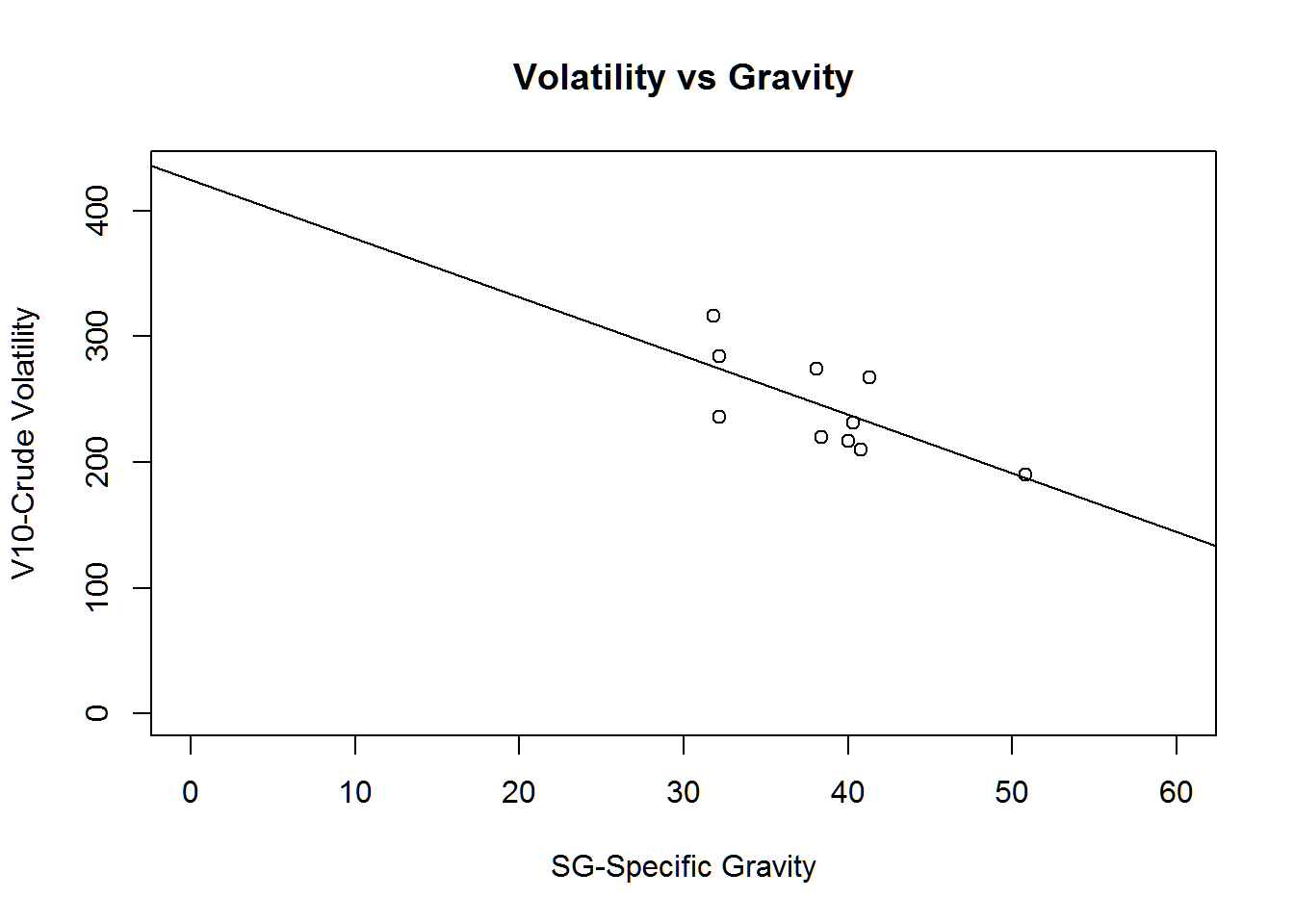regEP.Y <- lm(Y ~ EP, data = petrol) regEP.Y ## ## Call: ## lm(formula = Y ~ EP, data = petrol) ## ## Coefficients: ## (Intercept) EP ## -16.6621 0.1094 plot(petrol$EP, petrol\$Y, xlim = c(0, 450), ylim = c(-20, 50), xlab = 'EP-Volatility of Gas', ylab = 'Y-Yield', main = 'Yield vs Gas Volatility')
abline(regEP.Y)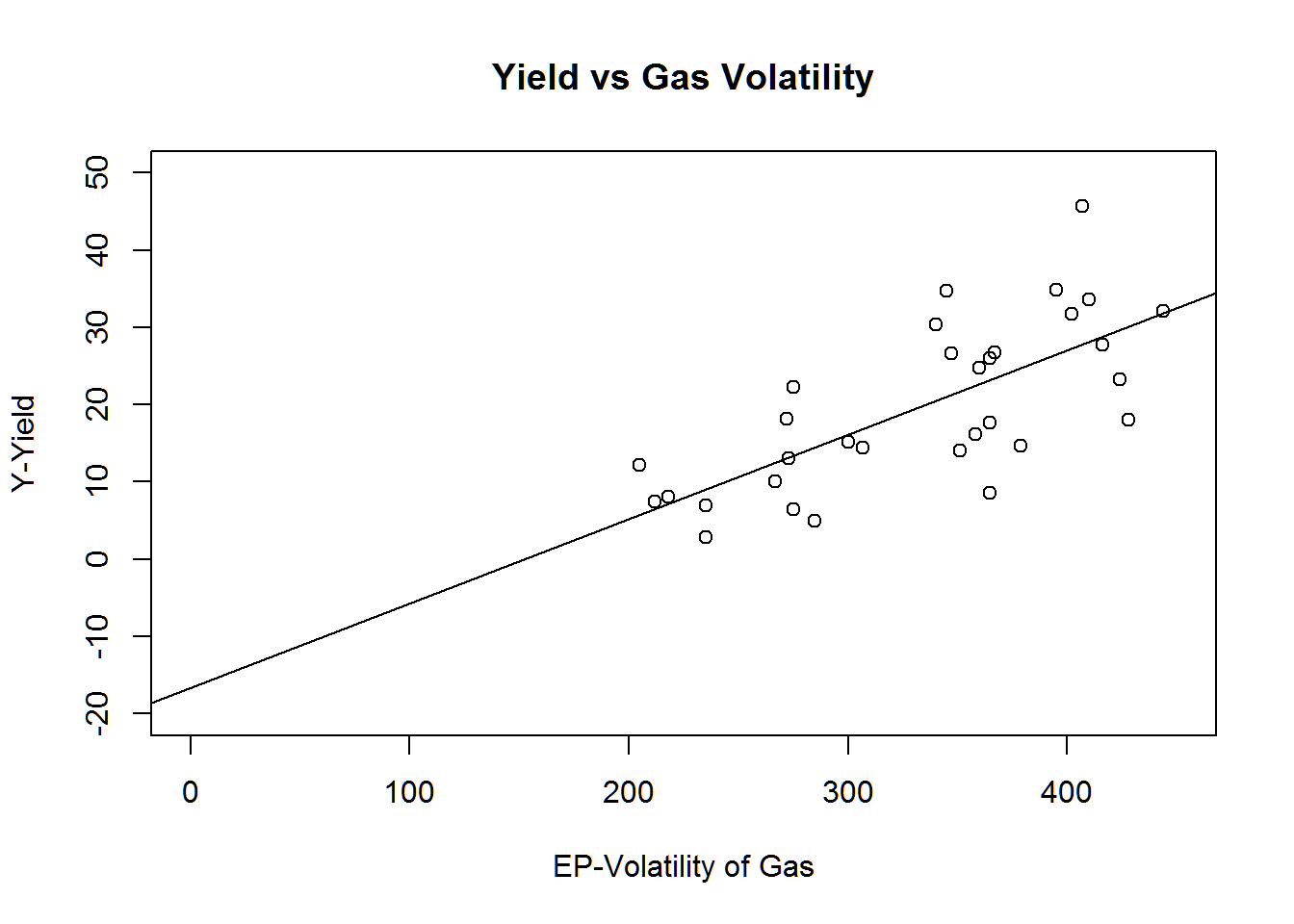It’s not necessary for us to examine each variable by only one variable at a time. We can use multivariate regression to test a single variable against the other.

There are methods to determine which variables are the most important to include in the multivariate regression model, but we won’t bother with that right now. We are just interested in creating a multivariate model.

There are different ways of doing this in R, but we will use the glm command. GLM stands for Generalized Linear Model.

m.reg.model <- glm(Y ~ SG + VP + V10 + EP, data = petrol, family = gaussian)
m.reg.model
##
## Call:  glm(formula = Y ~ SG + VP + V10 + EP, family = gaussian, data = petrol)
##
## Coefficients:
## (Intercept)           SG           VP          V10           EP
##     -6.8208       0.2272       0.5537      -0.1495       0.1547
##
## Degrees of Freedom: 31 Total (i.e. Null);  27 Residual
## Null Deviance:       3564
## Residual Deviance: 134.8     AIC: 148.8

Our equation is Yield = 0.227(SG) + 0.554(VP) -0.15(V10) + 0.155(EP) - 6.821. We can use the summary command to learn more about our analysis.

summary(m.reg.model)
##
## Call:
## glm(formula = Y ~ SG + VP + V10 + EP, family = gaussian, data = petrol)
##
## Deviance Residuals:
##     Min       1Q   Median       3Q      Max
## -3.5804  -1.5223  -0.1098   1.4237   4.6214
##
## Coefficients:
##              Estimate Std. Error t value Pr(>|t|)
## (Intercept) -6.820774  10.123152  -0.674   0.5062
## SG           0.227246   0.099937   2.274   0.0311 *
## VP           0.553726   0.369752   1.498   0.1458
## V10         -0.149536   0.029229  -5.116 2.23e-05 ***
## EP           0.154650   0.006446  23.992  < 2e-16 ***
## ---
## Signif. codes:  0 '***' 0.001 '**' 0.01 '*' 0.05 '.' 0.1 ' ' 1
##
## (Dispersion parameter for gaussian family taken to be 4.992739)
##
##     Null deviance: 3564.1  on 31  degrees of freedom
## Residual deviance:  134.8  on 27  degrees of freedom
## AIC: 148.83
##
## Number of Fisher Scoring iterations: 2